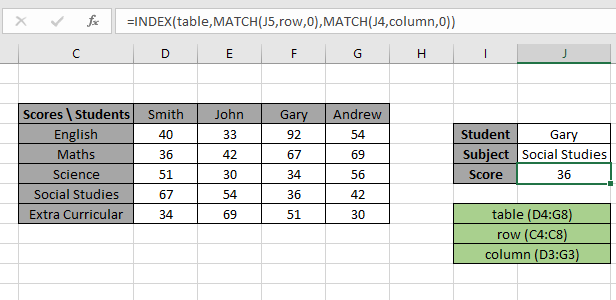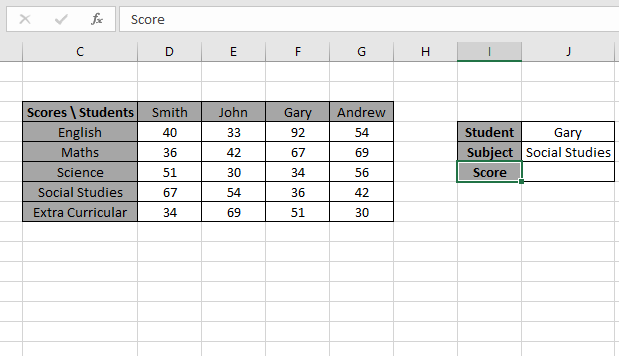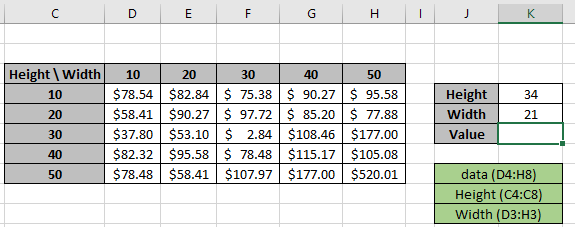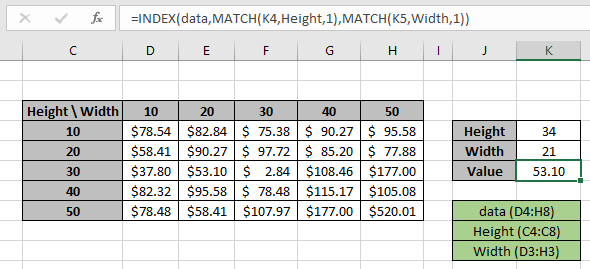# How to Do Two Way Lookup In Microsoft ExcelIn this article, we will learn How to Do Two Way Lookup In Microsoft Excel.

Scenario:

For instance, We need to find the value matched from the table without looking it up in the table. We need some fixed formula which helps in finding the exact match as required. 2D lookup table is the table where we need to match both row index and column index. For example, Finding Salary of an Employee (Emp 052). So the match function works twice one for Employee ID and other for Salary under Columns.

How to Solve the Problem?

For the formula to understand first we need to revise a little about the following functions

Formula

Now we will make a formula using the above functions. Match function will return the index of the lookup value1 in the row header field. And another MATCH function will returns the index of the lookup value2 in the column header field. The index numbers will now be fed into the INDEX function to get the values under the lookup value from the table data.

Generic Formula:

 = INDEX ( data , MATCH ( lookup_value1, row_headers, 0 , MATCH ( lookup_value2, column_headers, 0 ) ) )

data : array of values inside the table without headers

lookup_value1 : value to lookup in the row_header.

row_headers : Row Index array to lookup.

lookup_value1 : value to lookup in the column_header.

column_headers : column Index array to lookup.

Example:

The above statements can be complicated to understand. So let’s understand this by using the formula in an example

Here we have a list of scores gained by students with their Subject list. We need to find the Score for a specific Student (Gary) & Subject (Social Studies) as shown in the snapshot below.The Student value1 must match the Row_header array and Subject value2 must match the Column_header array.

Use the formula in the J6 cell:

 = INDEX ( table , MATCH ( J5, row, 0 , MATCH ( J4, column, 0 ) ) )

Explanation:

• The MATCH function matches the Student value in J4 cell with the row header array and returns its position 3 as a number.
• The MATCH function matches the Subject value in J5 cell with the column header array and returns its position 4 as a number.
• The INDEX function takes the row and column index number and looks up in the table data and returns the matched value.
• The MATCH type argument is fixed to 0. As the formula will extract the exact match.Here values to the formula is given as cell references and row_header, table and column_header given as named ranges.

As you can see in the above snapshot, we got the Score obtained by student Gary in Subject Social Studies as 36 .  And it proves the formula works fine and for doubts see the below notes for understanding.

Now we will use the approximate match with row headers and column headers as numbers. Approx match only takes the number values as there is no way it applies on text values

Here we have a price of values as per the Height & Width of the product. We need to find the Price for a specific Height (34) & Width (21) as shown in the snapshot below.The Height value1 must match the Row_header array and Width value2 must match the Column_header array.

Use the formula in the K6 cell:

 = INDEX ( data , MATCH ( K4, Height, 1 , MATCH ( K5, Width, 1 ) ) )

Explanation:

• The MATCH function matches the Height value in K4 cell with the row header array and returns its position 3 as a number.
• The MATCH function matches the Width value in K5 cell with the column header array and returns its position 2 as a number.
• The INDEX function takes the row and column index number and looks up in the table data and returns the matched value.
• The MATCH type argument is fixed to 1. As the formula will extract the approximate match.Here values to the formula is given as cell references and row_header, data and column_header given as named ranges as mentioned in the snapshot above.

As you can see in the above snapshot, we got the Price obtained by height (34) & Width (21) as 53.10 .  And it proves the formula works fine and for doubts see the below notes for more understanding.

Notes:

1. The function returns the #NA error if the lookup array argument to the MATCH function is 2 D array which is the header field of the data..
2. The function matches exact value as the match type argument to the MATCH function is 0.
3. The lookup values can be given as cell reference or directly using quote symbol ( " ) in the formula as arguments.

Hope this article about How to Do Two Way Lookup In Microsoft Excel is explanatory. Find more articles on looking up values and related Excel formulas here. If you liked our blogs, share it with your friends on Facebook. And also you can follow us on Twitter and Facebook. We would love to hear from you, do let us know how we can improve, complement or innovate our work and make it better for you. Write to us at info@exceltip.com.

Related Articles :

Use INDEX and MATCH to Lookup Value : INDEX & MATCH function to look up value as required.

SUM range with INDEX in Excel : Use INDEX function to find the SUM of the values as required.

How to use the SUM function in Excel : Find the SUM of numbers using the SUM function explained with example.

How to use the INDEX function in Excel : Find the INDEX of an array using the INDEX function explained with example.

How to use the MATCH function in Excel : Find the MATCH in the array using the INDEX value inside MATCH function explained with example.

How to use LOOKUP function in Excel : Find the lookup value in the array using the LOOKUP function explained with example.

Popular Articles :

How to use the IF Function in Excel : The IF statement in Excel checks the condition and returns a specific value if the condition is TRUE or returns another specific value if FALSE.

How to use the VLOOKUP Function in Excel : This is one of the most used and popular functions of excel that is used to lookup value from different ranges and sheets.

How to Use SUMIF Function in Excel : This is another dashboard essential function. This helps you sum up values on specific conditions.

How to use the COUNTIF Function in Excel : Count values with conditions using this amazing function. You don't need to filter your data to count specific values. Countif function is essential to prepare your dashboard.

Terms and Conditions of use

The applications/code on this site are distributed as is and without warranties or liability. In no event shall the owner of the copyrights, or the authors of the applications/code be liable for any loss of profit, any problems or any damage resulting from the use or evaluation of the applications/code.